# How to use the NORM.S.INV function in Excel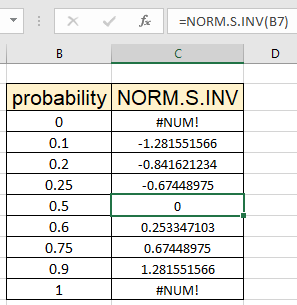In this article, we will learn How to use the NORM.S.INV function in Excel.

What is Normal Cumulative Distribution?

Normal Cumulative Distribution is a type of continuous probability distribution for a real-valued random variable. The general form of its probability density function is given by :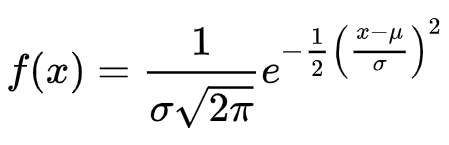The value is basically called the Z value. The given mathematical formula to calculate is very complex. So, You can calculate the normal cumulative distribution of the given specific mean and standard deviation for the dataset using the NORMDIST Function. Calculate the inverse of normal cumulative distribution using the NORM.INV Function.

Standard Normal Cumulative Distribution is a special case of the normal cumulative distribution with mean () = 0  and Standard deviation () = 1. The general form of Standard Normal Cumulative Distribution is given by :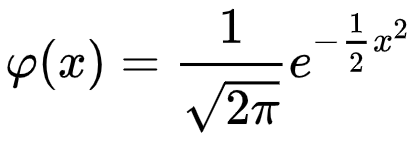You can calculate standard normal cumulative distribution using mean 0 and standard deviation 1 within NORMDIST Function in Excel but Inverse of the standard normal distribution computes the critical value so that the cumulative distribution is greater than or equal to a pre-specified probability value (x). Learn how to calculate the inverse of standard Normal Cumulative Distribution in Excel using the NORM.S.INV function. That is, it returns the inverse of Z value, given the cumulative probability.

The standard normal distribution has a mean of zero and a standard deviation of one. The probability density vs standard deviations curve for the standard normal cumulative distribution is given below.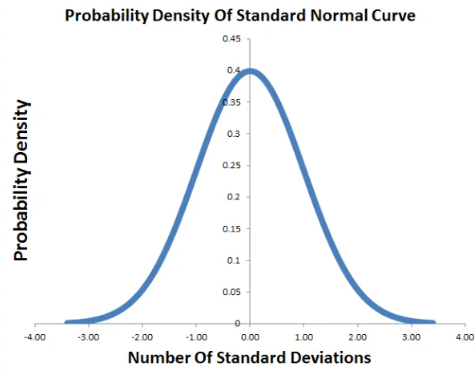NORM.S.INV Function in Excel

NORM.S.INV function returns the inverse of the standard normal cumulative distribution for the dataset. The function only takes one argument, the probability value of the dataset.

NORM.S.INV Function syntax:

 =NORM.S.INV(probability)

probability : probability value for the distribution dataset

Example :

All of these might be confusing to understand. Let's understand how to use the function using an example. Here we have a dataset first we calculate the probability for the dataset and then we will calculate the inverse of the normal cumulative distribution for the same.

Use the formula:

 =NORM.S.INV(0.1)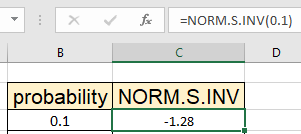This is the inverse of Z value for the given 0.1 probability having default mean 0 and standard deviation 1 comes out to be approx -1.28. Now you can calculate the inverse of Z value for the specified probability values.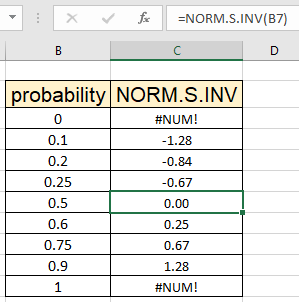You can supply the probability value directly or using the cell reference. As you inspect from the above image that inverse of Z value for probability 0.1 and 0.9 has the same magnitude but different signs.

If you want to learn more about normal cumulative distribution, click here. This will enhance your understanding of the function with a random variable dataset.

Here are all the observational notes using the NORM.S.INV function in Excel
Notes :

1. The function returns #VALUE! Error if the probability value is non-numeric
2. The function returns #NUM! Error if the probability is either <= 0 or >= 1. This error doesn't occur if you use the PROB function with NORM.S.INV function.

Hope this article about How to use the NORM.S.INV function in Excel is explanatory. Find more articles on statistical formulas and related Excel functions here. If you liked our blogs, share it with your friends on Facebook. And also you can follow us on Twitter and Facebook. We would love to hear from you, do let us know how we can improve, complement or innovate our work and make it better for you. Write to us at info@exceltip.com.

Related Articles :

How to use Excel NORM.DIST Function : Calculate the Z score for the normal cumulative distribution for the pre specified values using the NORMDIST function in Excel.

How to use Excel NORM.INV Function : Calculate the inverse of Z score for the normal cumulative distribution for the pre-specified probability values using the NORM.INV function in Excel.

How to Calculate Standard Deviation in Excel : To calculate the standard deviation we have different functions in Excel. The standard deviation is the square root of the variance value but It tells more about the dataset than variance.

Regressions Analysis in Excel : Regression is an Analysis Tool, which we use for analyzing large amounts of data and making forecasts and predictions in Microsoft Excel.

How to Create Standard Deviation Graph : The standard deviation tells how much data is clustered around the average of the data. Learn how to create a standard deviation graph here.

How to use the VAR function in Excel : Calculate the variance for the sample dataset in excel using the VAR function in Excel.

Popular Articles :

How to use the IF Function in Excel : The IF statement in Excel checks the condition and returns a specific value if the condition is TRUE or returns another specific value if FALSE.

How to use the VLOOKUP Function in Excel : This is one of the most used and popular functions of excel that is used to lookup value from different ranges and sheets.

How to use the SUMIF Function in Excel : This is another dashboard essential function. This helps you sum up values on specific conditions.

How to use the COUNTIF Function in Excel : Count values with conditions using this amazing function. You don't need to filter your data to count specific values. Countif function is essential to prepare your dashboard.

Terms and Conditions of use

The applications/code on this site are distributed as is and without warranties or liability. In no event shall the owner of the copyrights, or the authors of the applications/code be liable for any loss of profit, any problems or any damage resulting from the use or evaluation of the applications/code.